### Differential Equations I

Perhaps you're a financial advisor forecasting stock market prices, or maybe you're a biologist studying competing species.

There's a chance you're a chemist charting a reaction or an engineer designing a bridge.

It's possible that you're a physicist pushing the frontiers of cosmic knowledge or a mathematician in awe of the abstract.

No matter your role, you're bound to come across differential equations.

# Differential Equations in a Nutshell

In a nutshell, a differential equation involves unknown functions and derivatives.

$y'(x) = x^2/y(x)^2$ is a perfect example; it relates the unknown $y(x)$ to its first derivative $y'(x).$

In this course we'll use calculus to solve such problems and apply geometry to sketch solution curves (see below).

To start things off, let's take a quick tour of our most important topics!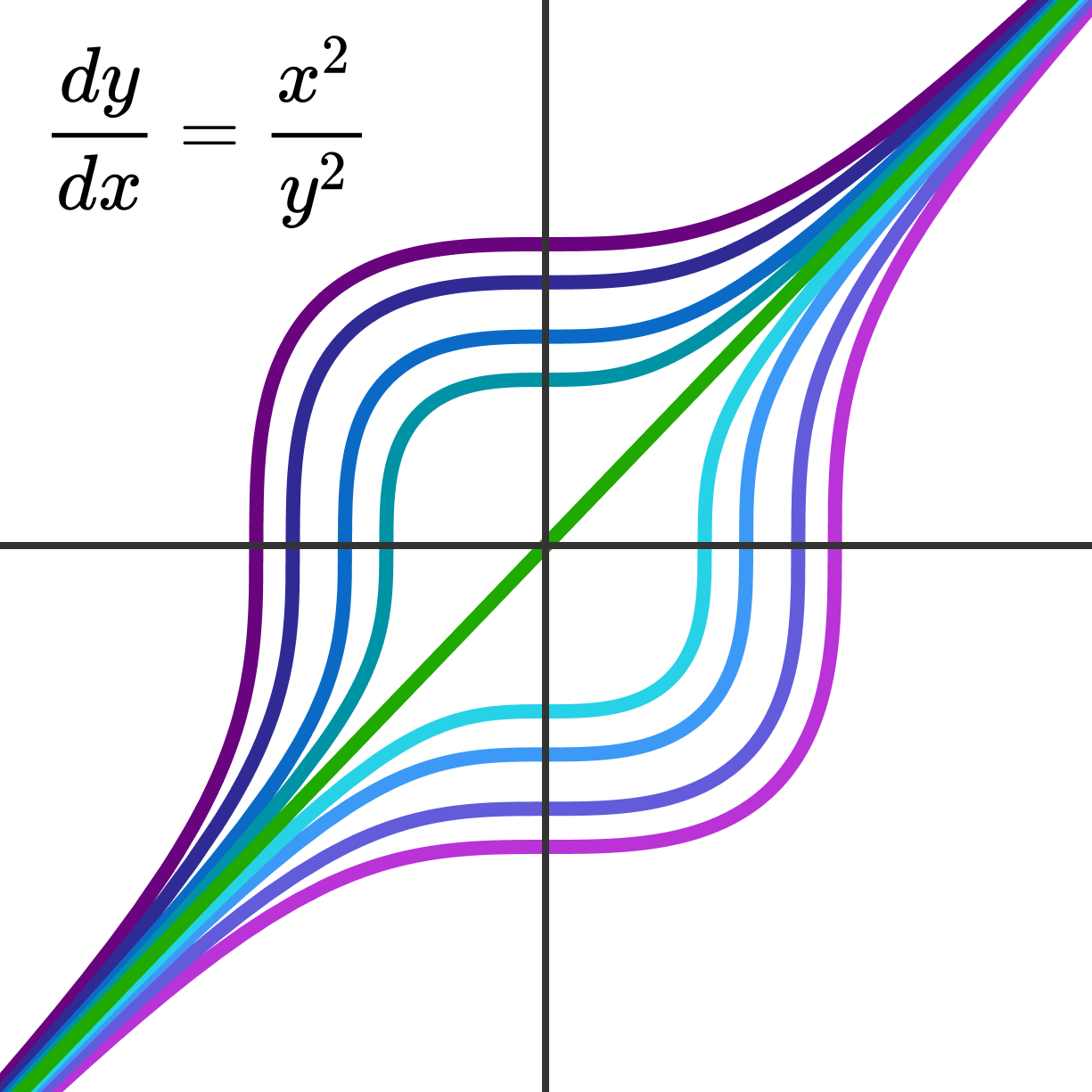Solution curves for $y'(x) = x^2/y(x)^2.$

# Differential Equations in a Nutshell

A solution to a differential equation is a function that makes it true, like $x=1$ is the solution of $x^2- 2 x + 1 = 0$ since $1^2-2\times 1+1 = 0.$

If you think about it, you already solved a bunch of differential equations just going through calculus!

A classic calculus problem is to find the area below a curve, like the one below.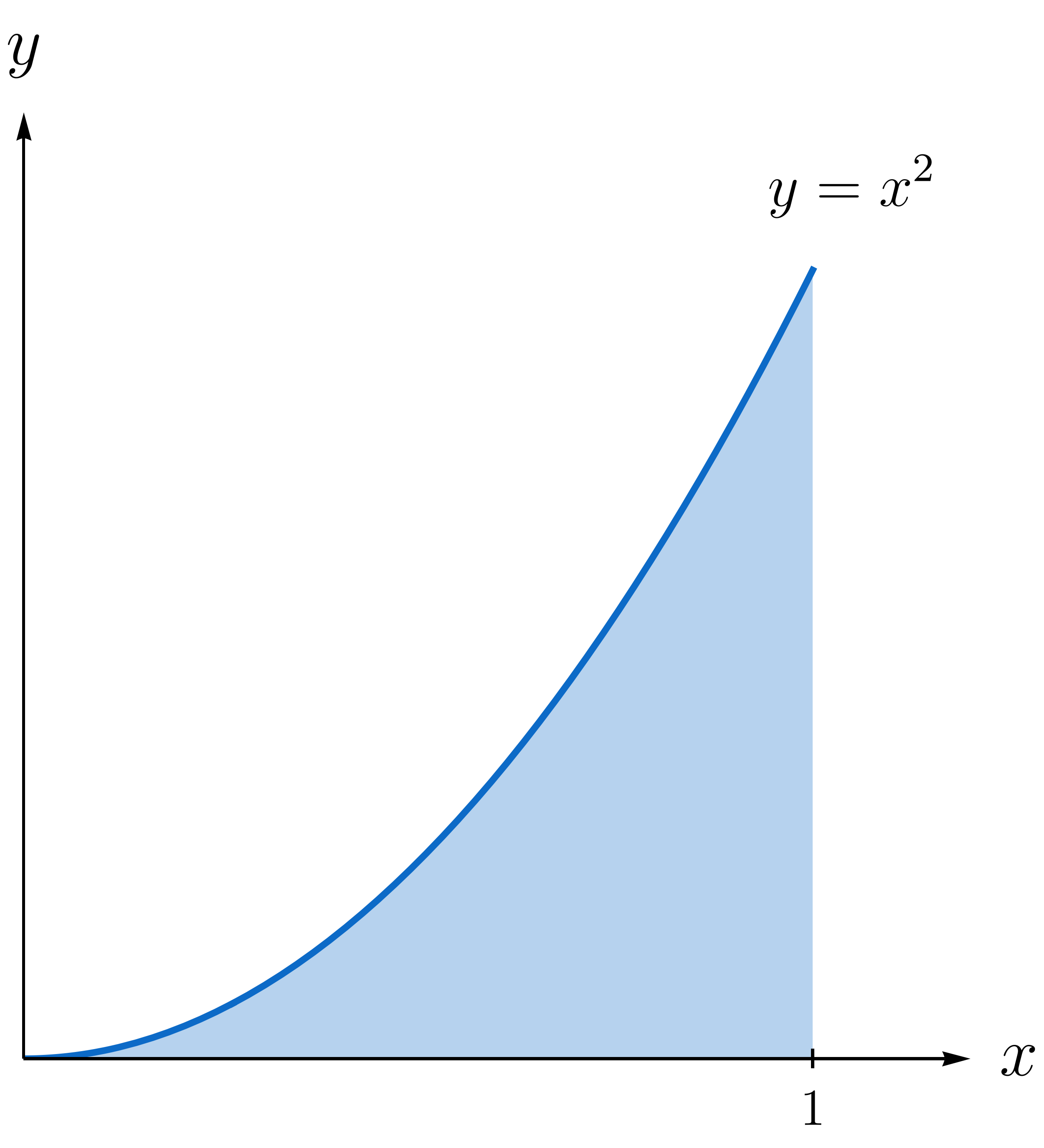It's given by $A = \int_{x=0}^{x=1} x^2 dx,$ and to compute this integral, we apply the fundamental theorem of calculus $\int\limits_{x=0}^{x=1}x^2 dx = F(1) - F(0), \ \ \text{where} \ \ \ F'(x) = x^2.$ So finding the area under the parabolic arc means solving the differential equation $F'(x) = x^2 .$

Solve this equation and find $F(x).$ In all the options, $C$ is a constant.

Hint: The power rule $\frac{d}{dx}[ x^{n} ] = n x^{n-1}$ is a good place to start.

# Differential Equations in a Nutshell

Here's another calculus classic: a ball is thrown straight down with speed $u$ from a wall $H$ units tall. The height of the ball $h(t)$ obeys $h''(t) = - g$ where $g$ is a constant acceleration due to gravity.We'd really like to know $h(t),$ but writing the differential equation as $\frac{d^2 h}{dt^2} = \frac{d}{dt} \left( \frac{d}{dt}\big[h(t)\big] \right) = -g,$ there are clearly two derivatives standing between us and $h(t).$

Luckily, calculus gives us the tool for “undoing” a derivative: the indefinite integral. Use $\int t^{n} dt = \frac{1}{n+1} t^{n+1} +C, n \neq -1$ to remove the derivatives on $h(t).$

Note: Be careful to remember where the ball starts and how fast it begins to fall to the ground! Also keep in mind that $h'(t)$ is the ball's velocity: negative $h'(t)$ means the height is decreasing, and the ball is headed downwards.

# Differential Equations in a Nutshell

Undetermined constants often pop up when solving a differential equation, which means that the equation alone is an incomplete picture. We need extra conditions to complete our model.

Conditions are just pieces of data needed to nail down the exact shape of a solution, as initial velocity and height were used to set $h(t)$ for the falling ball. It'd be nice to know in advance how many such constants to expect so we know how many conditions we need to fix them.

The order $n,$ the highest derivative appearing in the equation, is a good guide. For instance, $F'(x) = x^2$ is first-order $(n=1)$ and $F(x)$ has just the one constant, while $h(t),$ which obeys the second-order $(n=2)$ equation $h''(t) = - g$, has two constants in need of fixing.

# Differential Equations in a Nutshell

Let's get some practice predicting how many conditions we need to complete a differential equation solution.

Towards the end of our course, we'll help lift a sunken treasure off the floor of the sea using a winch driven by an electric motor.In order to know if the motor's battery has enough energy to lift the chest, we'll need to solve $\theta^{(3)}(t) + \theta''(t) -\theta'(t) - \theta(t) = b,$ where $b$ is a constant depending on the battery, and the unknown $\theta(t)$ measures the number of winch revolutions as a function of time $t.$

How many conditions do we expect to need in order to completely determine $\theta(t)?$

Notation: In general, $y^{(n)}(x) = \frac{d^n y}{dx^{n}}, \ y^{(0)}(x) = y(x).$

# Differential Equations in a Nutshell

A differential equation isn't limited to just one independent variable. In fact, the vibrating I-beam we'll encounter later in the course obeys $\frac{\partial^4 w}{\partial x^4} = - \frac{\partial^2 w}{\partial t^2},$ where $w(x,t)$ measures how much the beam is bent at a point $x$ along its length at time $t.$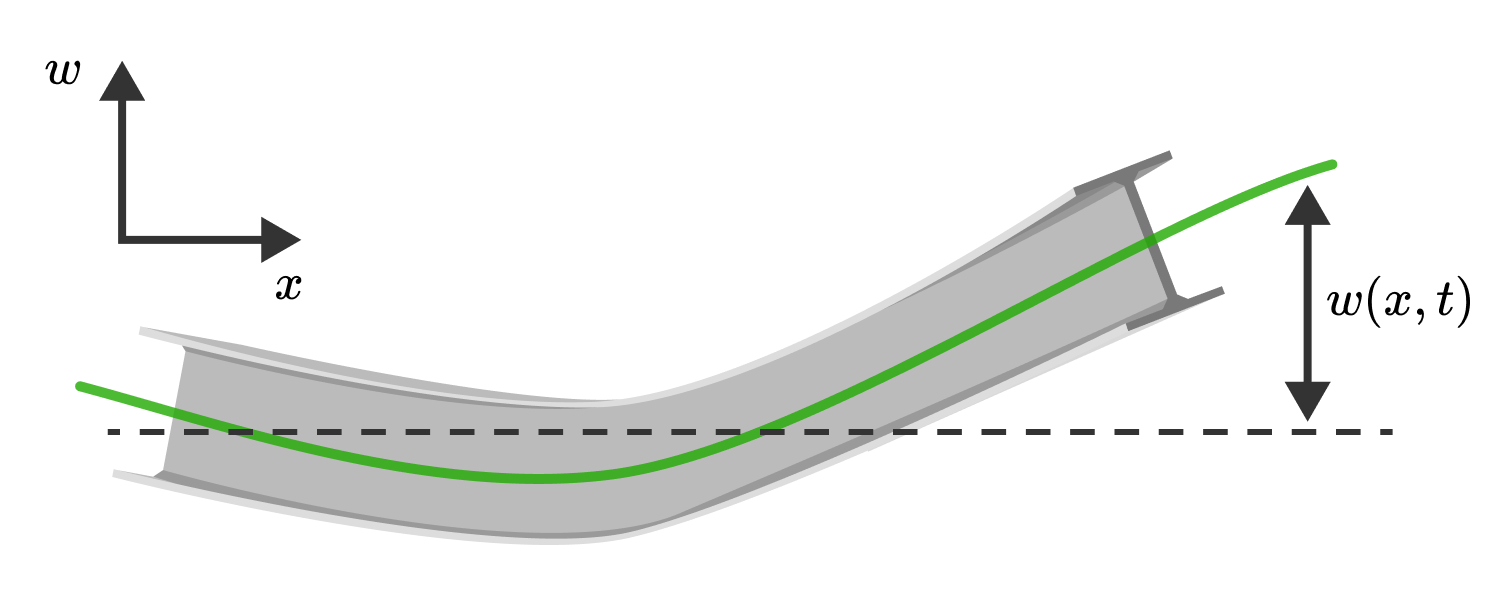I-beams support buildings and bridges, so knowing how vibrations from the outside world affect them is important to a structural engineer.

The equation $w_{xxxx} = - w_{tt}$ is one of the simplest vibrational models, and we'll tackle it head on once we have more experience.

# Differential Equations in a Nutshell

The I-beam equation on the last page is a partial differential equation since it involves two variables and their partial derivatives; it's fourth-order since there are more $x$ partial derivatives than there are $t.$Such an equation is a bit beyond our skill to crack right now, but that doesn't stop us from testing a given function to see if it solves the problem. Even when we can solve an equation directly, it's a good idea to plug our answer back in and see if everything checks out.

Among the options given, there's one that solves the I-beam equation $\frac{\partial^4 w}{\partial x^4} = - \frac{\partial^2 w}{\partial t^2}.$ Which one is it?

# Differential Equations in a Nutshell

The solution $w(x,t) =\sin(x) \sin(t)$ can be plotted as a surface.

While this can be very helpful in understanding its general behavior, it also gives us the perfect excuse to play around with one of several 3D touch-interactive plots sprinkled throughout the course!

The blue surface is the graph of $w(x,t),$ the green plane represents a constant time slice $t = \text{constant},$ and the red curve represents the shape of the beam at this time. You can rotate the perspective and zoom in and out with touch, and control the time $t$ through the slider.

In addition to 3D visualizations, we'll also use 2D interactives, like the one animating $w(x,t)$ (red) in time $t$ below.

# Differential Equations in a Nutshell

The I-beam equation shows us that it's sometimes necessary to have more than one independent variable, but it's also quite common to have more than one unknown function.

For example, a charged particle in a constant magnetic field $\vec{B}$ has a velocity vector $\vec{v}(t) = \big\langle v_{x}(t), v_{y}(t), v_{z}(t)\big\rangle,$ which obeys the system of equations \begin{aligned} \frac{dv_{x}}{dt} = \omega v_{y}, \ \frac{dv_{y}}{dt} = -\omega v_{x} , \ \frac{dv_{z}}{dt} = 0. \end{aligned} Notice that we don't have just three separate equations; the first two blend $v_{x}$ and $v_{y}$ together.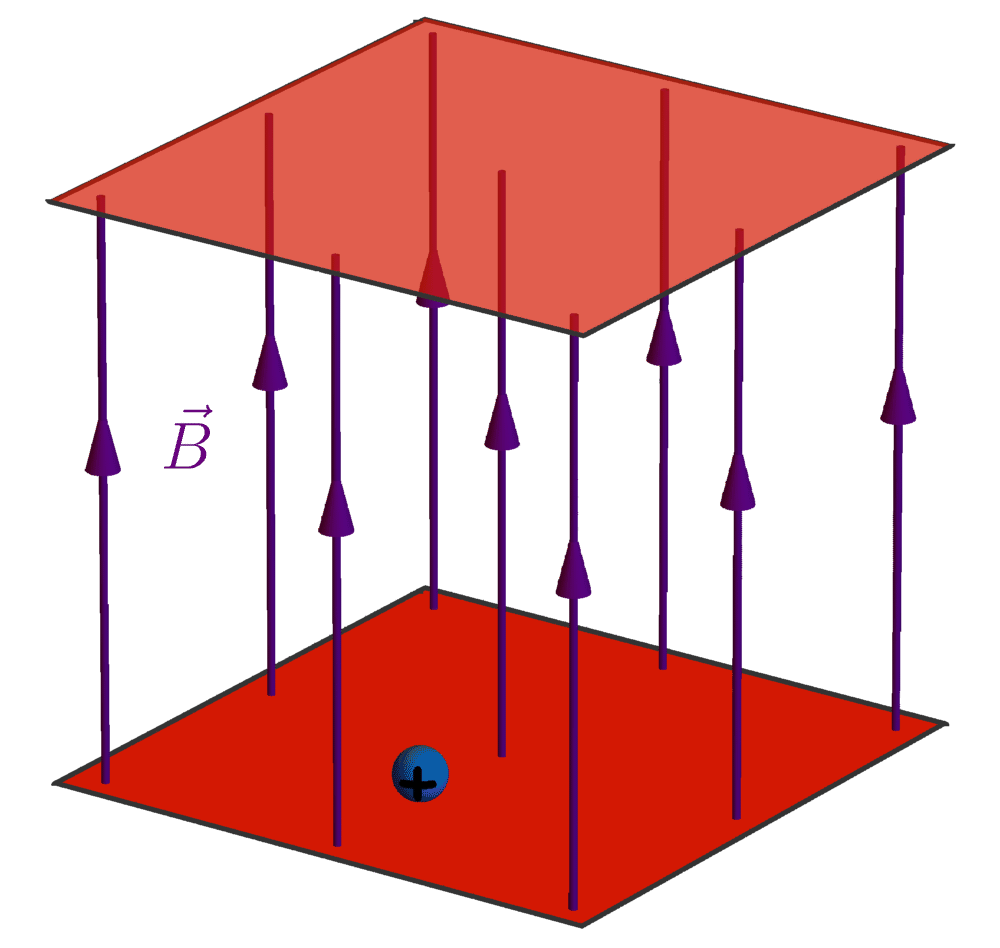We'll have a whole chapter set aside for such systems of equations, but as a sneak peek, a partial solution is given by \begin{aligned} v_{x}(t) & = v_{x}(0) \cos(\omega t) + v_{y}(0) \sin(\omega t) \\\\ v_{y}(t) & = \text{\_\_\_\_\_\_\_\_\_\_\_}+ v_{y}(0) \cos(\omega t) \\\\ v_{z}(t) & = v_{z}(0). \end{aligned} Here, $\vec{v}(0) = \big\langle v_{x}(0), v_{y}(0), v_{z}(0)\big\rangle$ is the initial velocity. The sine and cosine terms tell us that the particle circles around the $z$-axis, as in the animation.

Which option fills in the blank and completes the solution?

# Differential Equations in a Nutshell

“All happy families are alike; each unhappy family is unhappy in its own way.” - Tolstoy

This quote is apt for differential equations, though the division here is between linear and nonlinear types. For coefficients $a_i$ that at most depend on $t,$ a linear equation has the form $a_{n}(t) \frac{d^{n} y}{dt^{n}} + a_{n-1}(t) \frac{d^{n-1} y}{dt^{n-1}} + \dots + a_{0}(t) y = B(t).$ Here, $B(t)$ is a given function called the driving term. In short, a linear equation doesn't involve powers or products of $y$ and its derivatives.

Much of the first part of the course will go into solving linear equations with constant $a$'s: there's a clear and precise process that works to solve all such problems, which are common.

Solving a nonlinear equation involving products/quotients of $y$ and its derivatives is entirely different: when we can solve it (which is rare!), the method is usually specific to the equation.

But this doesn't mean nonlinear equations are weird things only a mathematician would care about: surprisingly many real-world models are nonlinear!

# Differential Equations in a NutshellThe Fitzhugh-Nagumo equation, for instance, is a relatively simple model of neuronal electrical activity.

Neurons relay information through the nervous system by creating an electrical potential $v(t)$ via a flow of sodium and potassium ions across the cell membrane.

The Fitzhugh-Nagumo model is a differential equation for this electrical potential: $\frac{dv}{dt} = v(t) - \frac{1}{3} v(t)^3.$ Is this equation linear or nonlinear?

# Differential Equations in a Nutshell

Nonlinear equations are not only hard to solve, but they're also quirky.

For example, the differential equation $y'(x) = \frac{x^2}{y(x)^2}$ is nonlinear, but it has known solutions: $y(x) =\sqrt{x^3+C}.$

Solutions are sometimes called integrals since integration plays a part in finding them; their graphs are called integral curves.

So $y'(x) = \frac{x^2}{y(x)^2}$ has infinitely many integral curves, one for every $C \in \mathbb{R}$; a few are pictured below.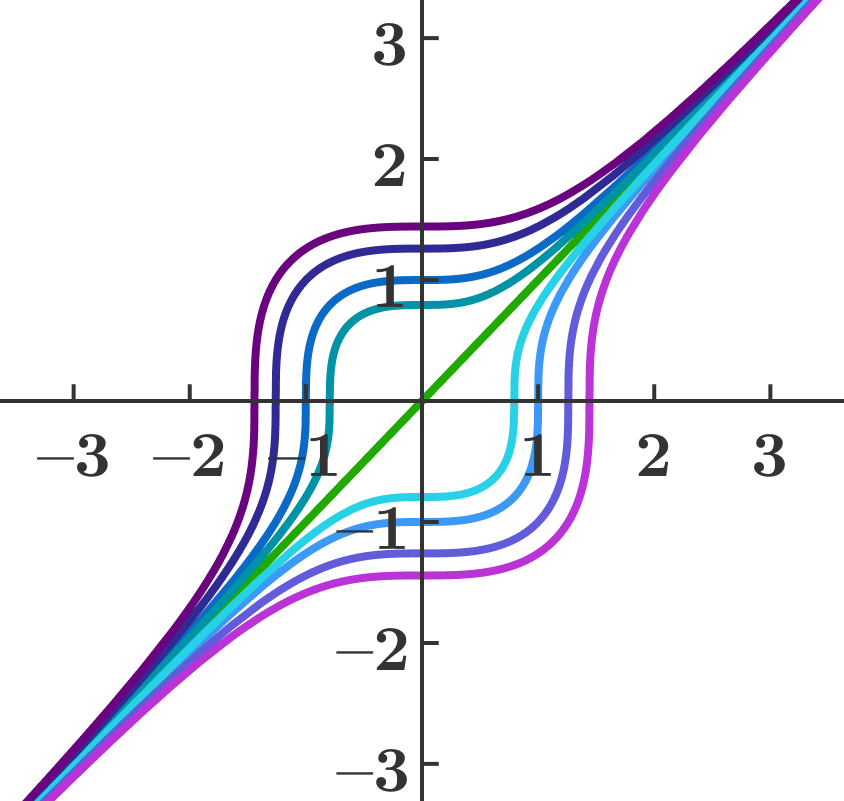No surprises yet since $y'(x) = \frac{x^2}{y(x)^2}$ is first-order, and the single undetermined constant $C$ is expected. But $|y'| + |y| = 0$ is also first-order and nonlinear thanks to the absolute value signs.

How many solutions (or integrals if you like that better) does this equation have?

# Differential Equations in a Nutshell

This whirlwind tour of differential equations covered a lot of ground, and there's much we have to do in later chapters to fill in the gaps.

It leaves us with two major questions, though: “Where do differential equations come from?” and, more importantly, “How do we solve one?”

The last question is going to take some work to fully address, but we can answer both in broad strokes with a simple model that you can discuss with friends over a cup of coffee.

Head on over to the next quiz where we'll build, analyze, and solve our very first differential equation!

# Differential Equations in a Nutshell

×